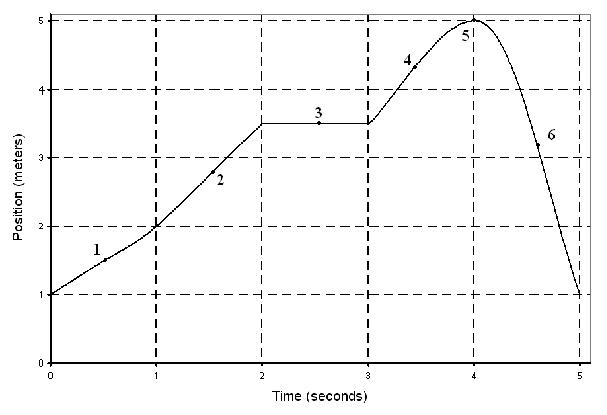# Problem: The speed at point 1 is less than the speed at point 2.a. Trueb. False

###### FREE Expert Solution

The slope of a position-time graph is the velocity.###### Problem DetailsThe speed at point 1 is less than the speed at point 2.

a. True

b. False